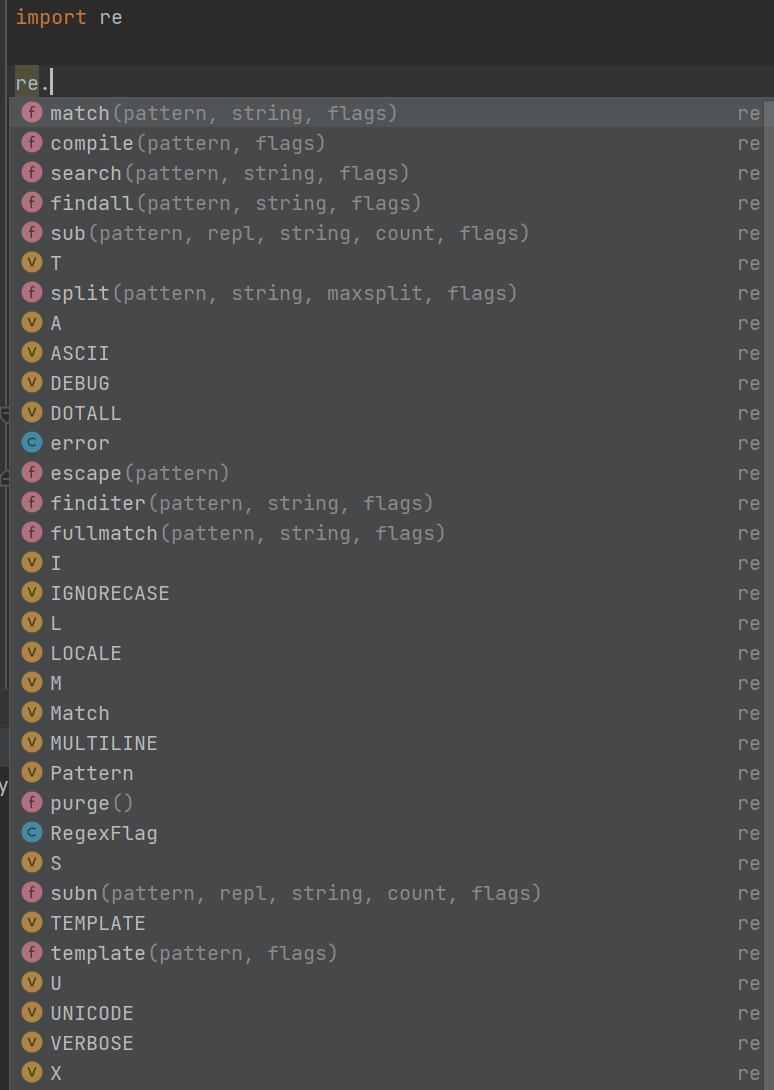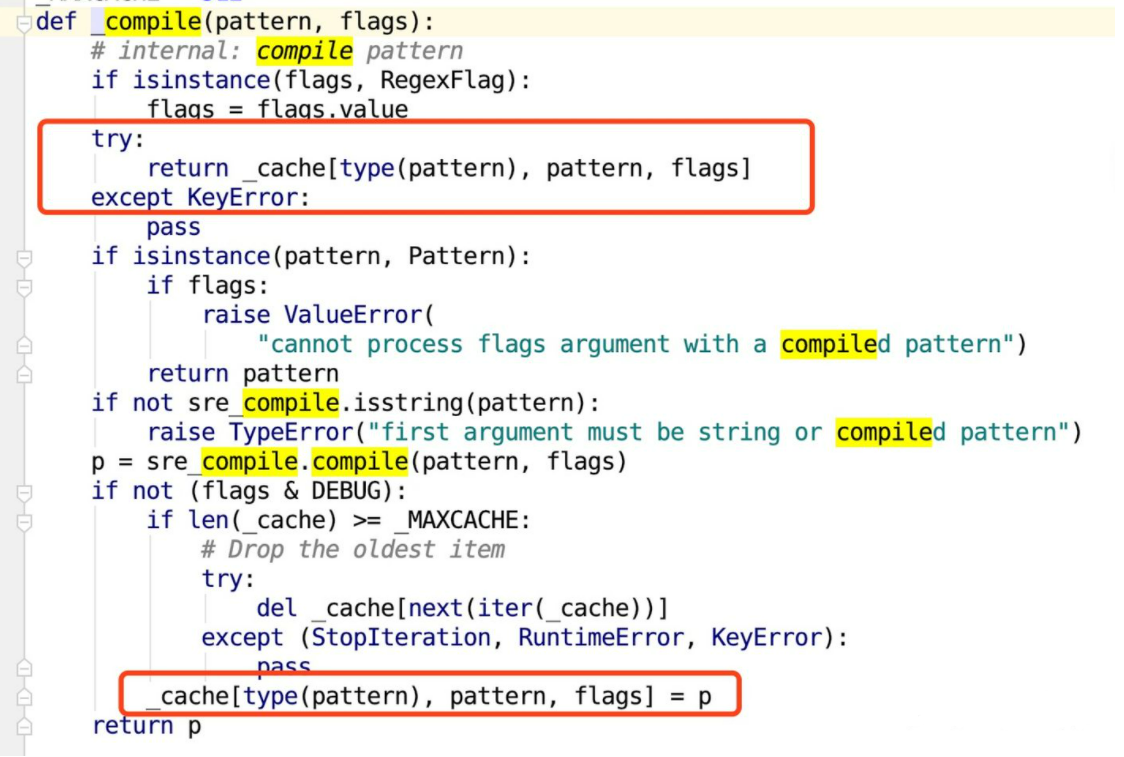# python中的re模块

Python中re模块主要功能是通过正则表达式是用来匹配处理字符串的

``````import re
``````

re有很多的方法和属性re 模块提供了不少有用的函数，用以匹配字符串，比如：

compile 函数
match 函数
search 函数
findall 函数
finditer 函数
split 函数
sub 函数
subn 函数
re 模块的一般使用步骤如下：

## compile 函数

compile 函数用于编译正则表达式，生成一个 Pattern 对象，它的一般使用形式如下：

``````re.compile(pattern,flag=0)
'''
pattern： 正则模型
falgs ： 匹配模式,比如忽略大小写，多行模式等

'''
``````

``````import re
# 将正则表达式编译成 Pattern 对象
pattern = re.compile(r'\d+')
``````

match 方法
search 方法
findall 方法
finditer 方法
split 方法
sub 方法
subn 方法

compile()的定义：

``````compile(pattern, flags=0)
Compile a regular expression pattern, returning a pattern object.
``````

### compile()与findall()一起使用，返回一个列表。

``````import re
def main():
content = 'Hello, I am Jerry, from Chongqing, a montain city, nice to meet you……'
regex = re.compile('\w*o\w*')
x = regex.findall(content)
print(x)
if __name__ == '__main__':
main()
# ['Hello', 'from', 'Chongqing', 'montain', 'to', 'you']
``````

### compile()与match()一起使用，可返回一个class、str、tuple，dict。

compile()与match()一起使用，可返回一个class、str、tuple，dict。 但是一定需要注意match()，从位置0开始匹配，匹配不到会返回None，返回None的时候就没有span/group属性了，并且与group使用，返回一个单词‘Hello’后匹配就会结束。

``````import re
def main():
content = 'Hello, I am Jerry, from Chongqing, a montain city, nice to meet you……'
regex = re.compile('\w*o\w*')
y = regex.match(content)
print(y)
print(type(y))
print(y.group())
print(y.span())
print(y.groupdict())

if __name__ == '__main__':
main()
# <_sre.SRE_Match object; span=(0, 5), match='Hello'>
# <class '_sre.SRE_Match'>
# Hello
# (0, 5)
# {}
``````

### compile()与search()搭配使用

compile()与search()搭配使用, 返回的类型与match()差不多， 但是不同的是search(), 可以不从位置0开始匹配。但是匹配一个单词之后，匹配和match()一样，匹配就会结束。

``````import re
def main():
content = 'Hello, I am Jerry, from Chongqing, a montain city, nice to meet you……'
regex = re.compile('\w*o\w*')
z = regex.search(content)
print(z)
print(type(z))
print(z.group())
print(z.span())
print(z.groupdict())
if __name__ == '__main__':
main()
# <_sre.SRE_Match object; span=(0, 5), match='Hello'>
# <class '_sre.SRE_Match'>
# Hello
# (0, 5)
# {}
``````

## 隐藏的 compile 函数

``````import re
pattern = re.compile('正则表达式')
text = '一段字符串'
result = pattern.findall(text)
``````

``````_compile(pattern, flag).对应的方法(string)
``````

``````def findall(pattern, string, flags=0):
"""Return a list of all non-overlapping matches in the string.

If one or more capturing groups are present in the pattern, return
a list of groups; this will be a list of tuples if the pattern
has more than one group.

Empty matches are included in the result."""
return _compile(pattern, flags).findall(string)
``````

``````def compile(pattern, flags=0):
"Compile a regular expression pattern, returning a Pattern object."
return _compile(pattern, flags)
``````

``````texts = [包含一百万个字符串的列表]
pattern = re.compile('正则表达式')
for text in texts:
pattern.search(text)
``````

``````texts = [包含一百万个字符串的列表]
for text in texts:
re.search('正则表达式', text)
``````## re中的正则匹配函数

### 1、match()函数（以后常用）

match，从头匹配一个符合规则的字符串，从起始位置开始匹配，匹配成功返回一个对象，未匹配成功返回None

``````match(pattern, string, flags=0)
# pattern： 正则模型
# string ： 要匹配的字符串
# falgs ： 匹配模式
``````
``````import  re
str="hello egon bcd egon lge egon acd 19"
r=re.match("h\w+",str) #match，从起始位置开始匹配，匹配成功返回一个对象，未匹配成功返回None,非字母，汉字，数字及下划线分割
print(r.group()) # 获取匹配到的所有结果，不管有没有分组将匹配到的全部拿出来
print(r.groups()) # 获取模型中匹配到的分组结果，只拿出匹配到的字符串中分组部分的结果
print(r.groupdict())  # 获取模型中匹配到的分组结果，只拿出匹配到的字符串中分组部分定义了key的组结果
# hello
# ()
# {}

r2=re.match("h(\w+)",str) #match，从起始位置开始匹配，匹配成功返回一个对象，未匹配成功返回None
print(r2.group())
print(r2.groups())
print(r2.groupdict())

# hello
# ('ello',)
# {}

r3=re.match("(?P<n1>h)(?P<n2>\w+)",str)  #?P<>定义组里匹配内容的key(键)，<>里面写key名称，值就是匹配到的内容
print(r3.group())
print(r3.groups())
print(r3.groupdict())

# hello
# ('h', 'ello')
# {'n1': 'h', 'n2': 'ello'}
``````

### 2、search()函数

search,浏览全部字符串，匹配第一符合规则的字符串，浏览整个字符串去匹配第一个，未匹配成功返回None

``````search(pattern, string, flags=0)
# pattern： 正则模型
# string ： 要匹配的字符串
# falgs ： 匹配模式
``````

``````import  re
str="hello egon bcd egon lge egon acd 19"
r=re.search("h\w+",str) #match，从起始位置开始匹配，匹配成功返回一个对象，未匹配成功返回None,非字母，汉字，数字及下划线分割
print(r.group()) # 获取匹配到的所有结果，不管有没有分组将匹配到的全部拿出来
print(r.groups()) # 获取模型中匹配到的分组结果，只拿出匹配到的字符串中分组部分的结果
print(r.groupdict())  # 获取模型中匹配到的分组结果，只拿出匹配到的字符串中分组部分定义了key的组结果

# hello
# ()
# {}

r2=re.search("h(\w+)",str) #match，从起始位置开始匹配，匹配成功返回一个对象，未匹配成功返回None
print(r2.group())
print(r2.groups())
print(r2.groupdict())

# hello
# ('ello',)
# {}

r3=re.search("(?P<n1>h)(?P<n2>\w+)",str)  #?P<>定义组里匹配内容的key(键)，<>里面写key名称，值就是匹配到的内容
print(r3.group())
print(r3.groups())
print(r3.groupdict())

# hello
# ('h', 'ello')
# {'n1': 'h', 'n2': 'ello'}
``````

### 3、findall()函数

``````findall(pattern, string, flags=0)
# pattern： 正则模型
# string ： 要匹配的字符串
# falgs ： 匹配模式
``````

``````'''

'''
import re
r=re.findall("\d+\w\d+","a2b3c4d5") #浏览全部字符串，匹配所有合规则的字符串，匹配到的字符串方到一个列表中
print(r)
# ['2b3', '4d5'] #匹配成功的字符串，不再参与下次匹配，所以3c4也符合规则但是没有匹配到
``````

``````'''

'''
import re
r=re.findall("","a2b3c4d5") #浏览全部字符串，匹配所有合规则的字符串，匹配到的字符串方到一个列表中
print(r)
# ['', '', '', '', '', '', '', '', ''] #如果没有写匹配规则，也就是空规则，返回的是一个比原始字符串多一位的空字符串列表，如上是8个字符，返回是9个空字符
``````

``````'''

'''
import re
r=re.findall("(ca)*","ca2b3caa4d5") #浏览全部字符串，匹配所有合规则的字符串，匹配到的字符串方到一个列表中
print(r)
# ['ca', '', '', '', 'ca', '', '', '', '', '']#用*号会匹配出空字符
``````

``````'''

'''
import re
r=re.findall("a\w+","ca2b3 caa4d5") #浏览全部字符串，匹配所有合规则的字符串，匹配到的字符串方到一个列表中
print(r)
# ['a2b3', 'aa4d5']#匹配所有合规则的字符串，匹配到的字符串放入列表
``````

``````'''

'''
import re
r=re.findall("a(\w+)","ca2b3 caa4d5") #有分组：只将匹配到的字符串里，组的部分放到列表里返回
print(r)
# ['2b3', 'a4d5']#返回匹配到组里的内容返回
``````

``````'''

'''
import re
r=re.findall("(a)(\w+)","ca2b3 caa4d5") #有多分组：只将匹配到的字符串里，组的部分放到一个元组中，最后将所有元组放到一个列表里返回
print(r)
# [('a', '2b3'), ('a', 'a4d5')]#返回的是多维数组
``````

``````'''

'''
import re
r=re.findall("(a)(\w+(b))","ca2b3 caa4b5") #分组中有分组：只将匹配到的字符串里，组的部分放到一个元组中，先将包含有组的组，看作一个整体也就是一个组，把这个整体组放入一个元组里，然后在把组里的组放入一个元组，最后将所有组放入一个列表返回
print(r)
# [('a', '2b', 'b'), ('a', 'a4b', 'b')]#返回的是多维数组
``````

?:在有分组的情况下findall()函数，不只拿分组里的字符串，拿所有匹配到的字符串，注意?:只用于不是返回正则对象的函数如findall()

``````'''
?:在有分组的情况下findall()函数，不只拿分组里的字符串，拿所有匹配到的字符串，注意?:只用于不是返回正则对象的函数如findall()
'''
import re
r=re.findall("a(?:\w+)","a2b3 a4b5 edd") #?:在有分组的情况下，不只拿分组里的字符串，拿所有匹配到的字符串，注意?:只用于不是返回正则对象的函数如findall()
print(r)
# ['a2b3', 'a4b5']
``````

### 4、split()函数

``````split(pattern, string, maxsplit=0, flags=0)
# pattern： 正则模型
# string ： 要匹配的字符串
# maxsplit：指定分割个数
# flags  ： 匹配模式
``````
``````import  re
print(r)
print(r2)

# ['sdf', 'fdf', 'sfsf', 'sff']
# ['sdf', 'fdf', 'sfsfafsff']
``````

### 5、 sub()函数

``````sub(pattern, repl, string, count=0, flags=0)
# pattern： 正则模型
# repl   ： 要替换的字符串
# string ： 要匹配的字符串
# count  ： 指定匹配个数
# flags  ： 匹配模式
``````
``````import  re
print(r)

# sdf替换fdf替换sfsf替换sff
``````

### 6、subn()函数

``````subn(pattern, repl, string, count=0, flags=0)
# pattern： 正则模型
# repl   ： 要替换的字符串
# string ： 要匹配的字符串
# count  ： 指定匹配个数
# flags  ： 匹配模式
``````
``````import  re